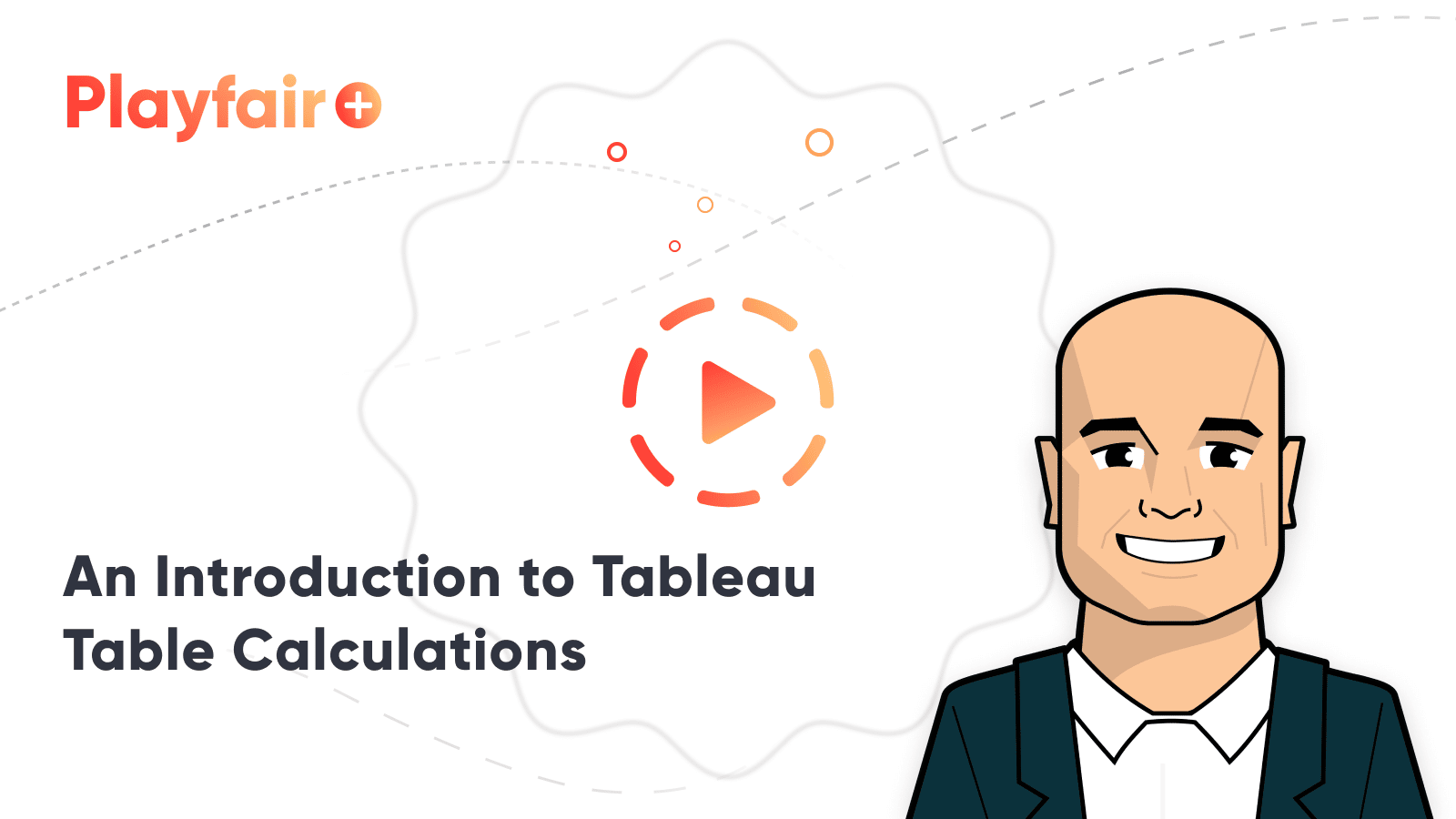# The Beginner’s Guide to Tableau Table Calculations

• Engineering
• Intermediate
• Tableau Desktop
• Technical Features

Tableau’s table calculations, while very powerful, can be both tricky and confusing to work with. There are some great benefits to using table calculations including efficient processing, quick calculations without needing to know the complex syntax, and much more. To properly utilize table calculations, the developer must have a firm understanding of how a table calculation behaves. This tutorial works as a guide to visually represent how different table calculation functions behave on a virtual data table.

Practical Tableau: An Introduction to Table Calculations

## Virtual data table

Behind every visualization lies a virtual data table, and the primary function of a table calculation is to partition through this data table. Table calculations operate first by the specified dimension, then by the direction in which the calculation needs to be run. Because these calculations are computed based on a virtual data table, they are restricted by what is in that table. For this reason, it is easiest to understand the behavior of a table calculation by visualizing it on a text table or crosstab prior to using it in a graph or other data visualization.

## Three parts of a table calculation

Every table calculation contains three separate parts.

1. The Measure
2. The Partitioning field

The Measure is the field that is being calculated using the table calculation. The easiest way to implement a table calculation is to right-click the relevant measure in the view and select “Quick Table Calculation” where there will be readily available options for you to choose from. These functions will get a table calc newbie pretty far in most circumstances and will help familiarize yourself with how table calculations behave before trying to write more advanced logic. When the quick table calculation has been applied to your measure, a delta symbol will appear in the pill.

The Partitioning field is a dimension that determines the boundaries of the table calculation. In other words, it defines what panes (if any) of the table that the calculation will run before restarting the calculation. For example, if region and state are dimensions in your table and region is the partitioning field (setting the boundaries), then the table calculation will run the calculation separately for each region in the table.

The Addressing fields are the dimensions that determine the direction which the table calculation runs. Tableau has some easy-to-use options available by right-clicking the measure and selecting ‘Compute Using’. These available options allow you to determine which direction you want your calculation to run (Table Down, Table Across, etc.).

As your table calculations grow more complex, you may have to bypass the easy to use ‘Compute Using’ options and manually define your addressing and partitioning fields using ‘Specific Dimensions’ which can be found by right-clicking the measure, selecting ‘Edit Table Calculation’, then choosing ‘Specific Dimensions’ under ‘Compute Using’.

The check marked dimensions become the addressing fields and any unchecked boxes become the partitioning field. For example, if your dimensions are Region and State and you want your table calculation to be calculated separately for each region, then you need to define Region as the partitioning field. This can be done by checking the State box to become the addressing field and leaving Region unchecked, making it the partitioning field.

## ‘Compute Using’ visual glossary

I have created the series of GIFs below to help our more visual learners, like myself, understand the behaviors of different table calculation functions.

There are many more table calculation applications and functions available in Tableau, but I hope that this visual guide will help jump start your understanding of the basics of how table calculations work. As always, reach out if you have any questions about this tutorial or if you have suggestions for any future content.

Thanks,

Dan Bunker
Visual Analytics Associate

## Become a memberAn Introduction to Tableau Table Calculations

## Related Content

### How to Color Entire Tableau Charts Based on Current PerformanceRyan Sleeper

Encode Entire Trend Charts with a Single Comparison Have you seen stock ticker gauges that show a full trend line…

### How to Highlight the Highest and Lowest Points in Tableau

This content is excerpted from my book, Innovative Tableau: 100 More Tips, Tutorials, and Strategies, published by O’Reilly Media Inc.,…

### How to Make Spark Bars with MIN MAX Indicators in TableauRyan Sleeper

Rounded Trend Bars, Custom Color Indicators, and a Labels Trick You’ll learn how to use the generated Measure Values field…